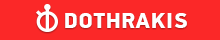GMAT Question of the Day - Daily to your Mailbox; hard ones only

 It is currently 26 Jun 2019, 11:22GMAT Club Daily Prep

Thank you for using the timer - this advanced tool can estimate your performance and suggest more practice questions. We have subscribed you to Daily Prep Questions via email.

Customized
for You

we will pick new questions that match your level based on your Timer History

Track

every week, we’ll send you an estimated GMAT score based on your performance

Practice
Pays

we will pick new questions that match your level based on your Timer History

Not interested in getting valuable practice questions and articles delivered to your email? No problem, unsubscribe here.In the figure above, O is the center of the circle. If AB has a length

Author Message
TAGS:

Hide Tags

Math ExpertV
Joined: 02 Sep 2009
Posts: 55803
In the figure above, O is the center of the circle. If AB has a length  [#permalink]

Show Tags00:00

Difficulty:25% (medium)

Question Stats:94% (01:23) correct6% (01:43) wrongbased on 24 sessions

HideShow timer StatisticsIn the figure above, O is the center of the circle. If AB has a length of 16 and OB has a length of 10, what is the length of CD ?

A. 2

B. 4

C. $$2\sqrt{3}$$

D. $$8-\sqrt{35}$$

E. $$8-\sqrt{39}$$

Attachment:2019-01-10_1419.png [ 16.83 KiB | Viewed 384 times ]

_________________
CEOD
Status: GMATINSIGHT Tutor
Joined: 08 Jul 2010
Posts: 2945
Location: India
GMAT: INSIGHT
Schools: Darden '21
WE: Education (Education)
Re: In the figure above, O is the center of the circle. If AB has a length  [#permalink]

Show Tags

Bunuel wrote:In the figure above, O is the center of the circle. If AB has a length of 16 and OB has a length of 10, what is the length of CD ?

A. 2

B. 4

C. $$2\sqrt{3}$$

D. $$8-\sqrt{35}$$

E. $$8-\sqrt{39}$$

In right triangle OBD, BD = (1/2)*AB = 8, AB = 10

Attachment:
2019-01-10_1419.png

i.e. OD = 6 (Using pythagorean triplet 6-8-10)

CD = CO - OD = 10-6 = 4

_________________
Prosper!!!
GMATinsight
Bhoopendra Singh and Dr.Sushma Jha
e-mail: info@GMATinsight.com I Call us : +91-9999687183 / 9891333772
Online One-on-One Skype based classes and Classroom Coaching in South and West Delhi
http://www.GMATinsight.com/testimonials.html

ACCESS FREE GMAT TESTS HERE:22 ONLINE FREE (FULL LENGTH) GMAT CAT (PRACTICE TESTS) LINK COLLECTION
InternB
Joined: 10 Feb 2018
Posts: 33
GMAT 1: 550 Q46 V20Re: In the figure above, O is the center of the circle. If AB has a length  [#permalink]

Show Tags

my answer is option B ie 4
OBD being a right angled triangle with OB =10 and BD=8, we get OD=6.
Therefore, CD=10-6=4
e-GMAT RepresentativeD
Joined: 04 Jan 2015
Posts: 2909
Re: In the figure above, O is the center of the circle. If AB has a length  [#permalink]

Show Tags

Solution

Given:
• O is the center of the circle
• AB = 16
• OB = 10

To find:
• The length of CD

Approach and Working:
OD bisects AB
• Thus, $$DB = \frac{AB}{2} = \frac{16}{2} = 8$$

In triangle ODB,
• $$OB^2 = OD^2 + DB^2$$
• Implies, $$100 = OD^2 + 64$$
• OD = 6

OC = radius = OD + CD
• CD = 10 – 6 = 4

Hence, the correct answer is Option B

_________________
CEOP
Joined: 18 Aug 2017
Posts: 3947
Location: India
Concentration: Sustainability, Marketing
GPA: 4
WE: Marketing (Energy and Utilities)
Re: In the figure above, O is the center of the circle. If AB has a length  [#permalink]

Show Tags

[quote="Bunuel"]In the figure above, O is the center of the circle. If AB has a length of 16 and OB has a length of 10, what is the length of CD ?

A. 2

B. 4

C. $$2\sqrt{3}$$

D. $$8-\sqrt{35}$$

E. $$8-\sqrt{39}$$

oc=ob=oa=10
and od=6 triangle ado = 3:4:5 = 6:8:10
so dc= oc-od = 10-6 = 4
IMO B
_________________
If you liked my solution then please give Kudos. Kudos encourage active discussions.Re: In the figure above, O is the center of the circle. If AB has a length   [#permalink] 10 Jan 2019, 07:42
Display posts from previous: Sort by

In the figure above, O is the center of the circle. If AB has a length